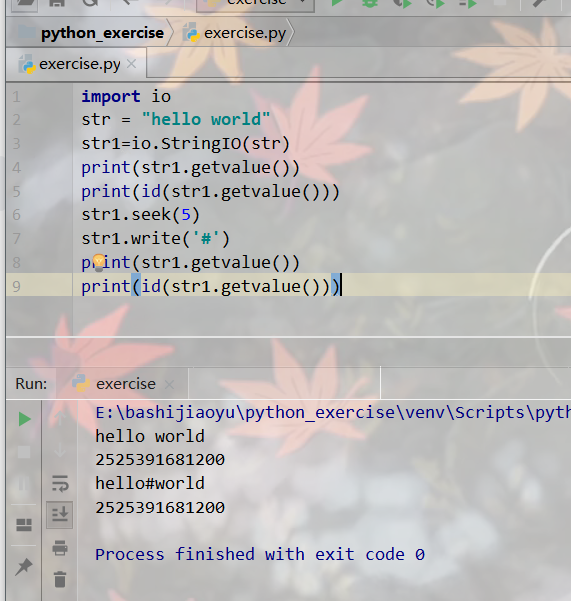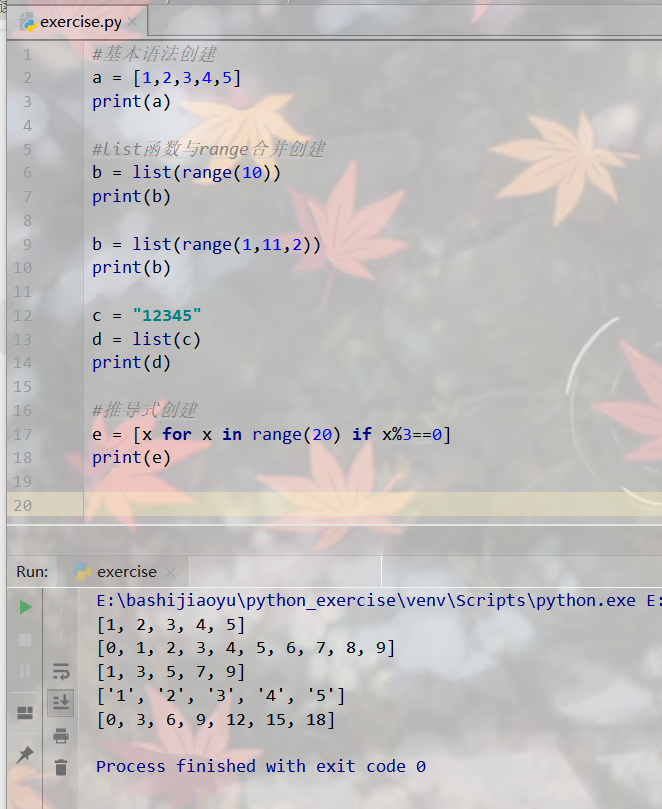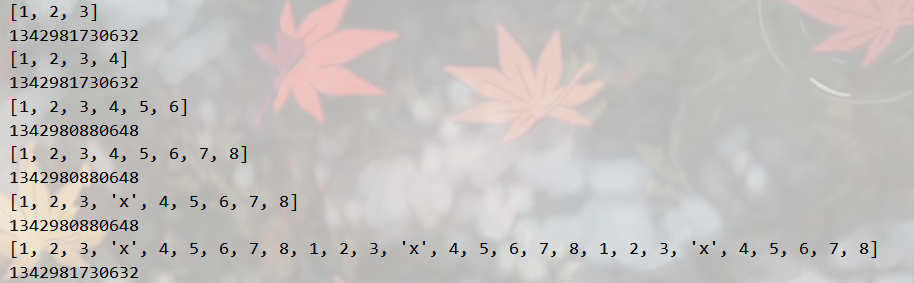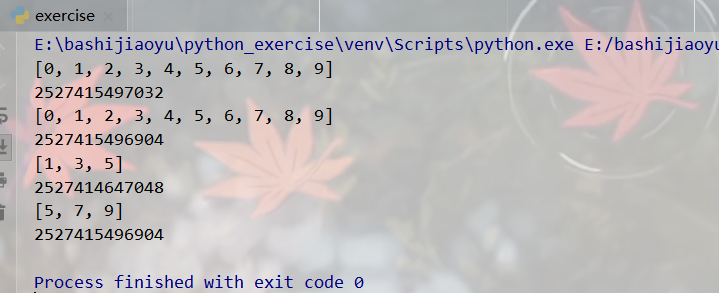Python基础笔记（三）原对象修改字符串、序列、列表、元组等1456-张同学

热门标签

为了实现原对象修改字符串，可以使用io.StringIO对象：

import io
str = "hello world"
str1=io.StringIO(str)
print(str1.getvalue())
print(id(str1.getvalue()))
str1.seek(5)
str1.write('#')
print(str1.getvalue())
print(id(str1.getvalue()))列表的创建：

#基本语法创建
a = [1,2,3,4,5]
print(a)

#list函数与range合并创建
b = list(range(10))
print(b)

b = list(range(1,11,2))
print(b)

c = "12345"
d = list(c)
print(d)

#推导式创建
e = [x for x in range(20) if x%3==0]
print(e)列表添加元素：append方法，'+'运算符，extend方法，insert方法，'*'运算符，其中'+'运算符和'*'运算符都会产生新的列表对象

a = [1,2,3]
print(a)
print(id(a))

a.append(4)
print(a)
print(id(a))

a = a + [5,6]
print(a)
print(id(a))

a.extend([7,8])
print(a)
print(id(a))

a.insert(3, 'x')
print(a)
print(id(a))

a = a*3
print(a)
print(id(a))偏移量小于 0 则会当做 0，终止偏移量大于“长度-1”会被当成”长度-1”。

a = [0,1,2,3,4,5,6,7,8,9]
print(a)
print(id(a))

#可以看到切片产生新的对象了
b = a[:]
print(b)
print(id(b))

b = a[1:7:2]
print(b)
print(id(b))

#负数表示倒数，-5表示倒数第5个
b = a[-5::2]
print(b)
print(id(b))评论 抢沙发觉得文章有用就打赏一下文章作者

支付宝扫一扫打赏微信扫一扫打赏Vieu3.3主题Q Q 登 录